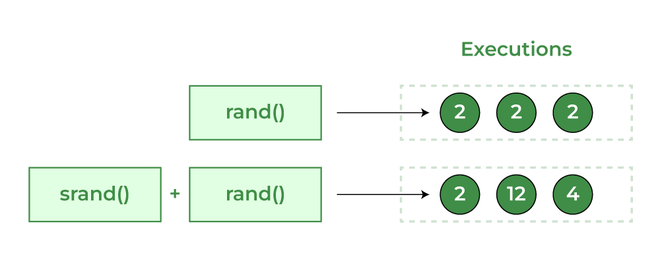GeeksforGeeks App
Open AppBrowser
Continue

# rand() and srand() in C++

rand() function is an inbuilt function in C++ STL, which is defined in header file <cstdlib>. rand() is used to generate a series of random numbers. The random number is generated by using an algorithm that gives a series of non-related numbers whenever this function is called. The rand() function is used in C++ to generate random numbers in the range [0, RAND_MAX).

RAND_MAX: It is a constant whose default value may vary between implementations but it is granted to be at least 32767.

### Syntax of rand()

`int rand(void);`

### Parameters of rand()

• This function does not take any parameters.

### Return Value of rand()

• rand() returns a pseudo-random number in the range of [0, RAND_MAX).

Say, we are generating 5 random numbers in C++ with the help of rand() in a loop, then every time we compile and run the program our output must be the same sequence of numbers.

## C++

 `// C++ program to demonstrate``//  the use of rand()``#include ``#include ``using` `namespace` `std;`` ` `int` `main()``{``    ``// This program will create some sequence of``    ``// random numbers on every program run``    ``for` `(``int` `i = 0; i < 5; i++)``        ``cout << ``rand``() << ``" "``;`` ` `    ``return` `0;``}`

Output

`1804289383 846930886 1681692777 1714636915 1957747793 `

### Complexity of rand() function

The time complexity and space complexity of the rand() function are as follows:

• Time Complexity: O(1)
• Auxiliary Space Complexity: O(1)

Note: This program will create same sequence of random numbers on every program run.

## C++

 `// C++ program to demonstrate the``// use of rand() to get value``// in a range of 0 to N-1``#include ``#include ``using` `namespace` `std;`` ` `int` `main()``{``    ``int` `N = 100;``    ``// This program will create some sequence of random``    ``// numbers on every program run within range 0 to N-1``    ``for` `(``int` `i = 0; i < 5; i++)``        ``cout << ``rand``() % N << ``" "``;`` ` `    ``return` `0;``}`` ` `// This code is contributed by Susobhan Akhuli`

Output

`83 86 77 15 93 `

## C++

 `// C++ program to demonstrate ``// the use of rand() to get value``// in a range of lb to ub``#include ``#include ``using` `namespace` `std;`` ` `int` `main()``{``    ``int` `lb = 20, ub = 100;``    ``// This program will create some sequence of random``    ``// numbers on every program run within range lb to ub``    ``for` `(``int` `i = 0; i < 5; i++)``        ``cout << (``rand``() % (ub - lb + 1)) + lb << ``" "``;`` ` `    ``return` `0;``}`` ` `// This code is contributed by Susobhan Akhuli`

Output

`66 90 38 99 88 `

## srand()

srand() function is an inbuilt function in C++ STL,  which is defined in <cstdlib> header file. srand() is used to initialize random number generators. The srand() function sets the starting point for producing a series of pseudo-random integers. If srand() is not called, the rand() seed is set as if srand(1) were called at the program start. Any other value for seed sets the generator to a different starting point.

### Syntax of srand()

```void srand( unsigned seed );
OR
int srand( unsigned int seed);```

Seeds the pseudo-random number generator used by rand() with the value seed.

### Parameters

• seed: A seed for a new sequence of pseudo-random numbers to be returned by successive calls to rand()

### Return value

• This function returns a pseudo-generated random number.

Note: The pseudo-random number generator should only be seeded once, before any calls to rand(), and at the start of the program. It should not be repeatedly seeded or reseeded every time you wish to generate a new batch of pseudo-random numbers.

Standard practice is to use the result of a call to srand(time(0)) as the seed. However, time() returns a time_t value which varies every time and hence the pseudo-random number varies for every program call.rand() and srand() comparison

## C++

 `// C++ program to generate random numbers``#include ``#include ``#include ``using` `namespace` `std;`` ` `int` `main()``{``    ``// This program will create different sequence of``    ``// random numbers on every program run`` ` `    ``// Use current time as seed for random generator``    ``srand``(``time``(0));`` ` `    ``for` `(``int` `i = 0; i < 4; i++)``        ``cout << ``rand``() << ``" "``;`` ` `    ``return` `0;``}`

Output

`1326865685 1413967981 1967280748 919663823 `

Time complexity: O(N), where N is the number of random numbers to be generated.

Note: This program will create a different sequence of random numbers on every program run.

## How srand() and rand() are related to each other?

srand() sets the seed which is used by rand to generate “random” numbers. If you don’t call srand before your first call to rand, it’s as if you had called srand(1) to set the seed to one.
In short, srand() — Set Seed for rand() Function

My Personal Notes arrow_drop_up